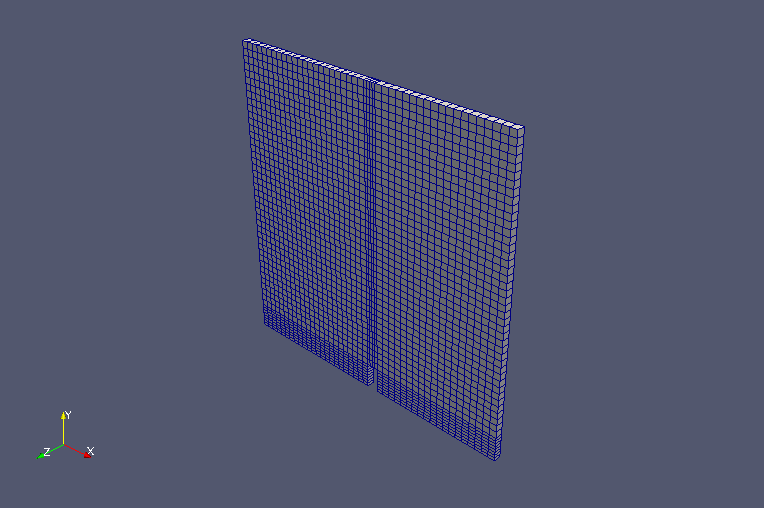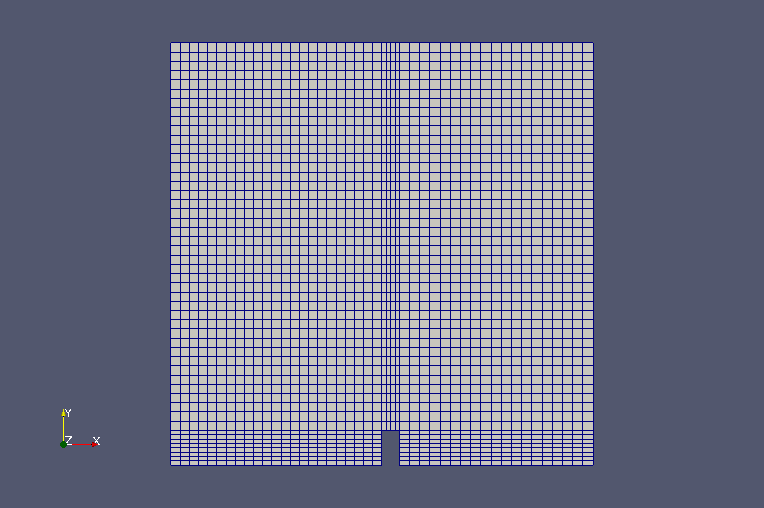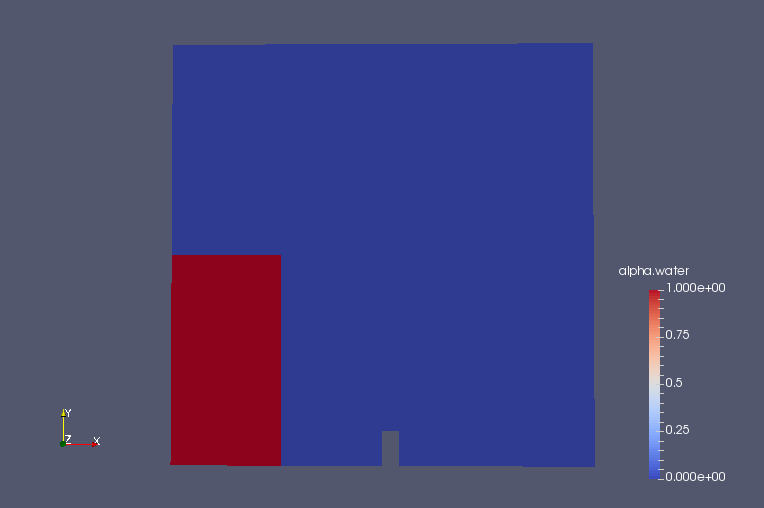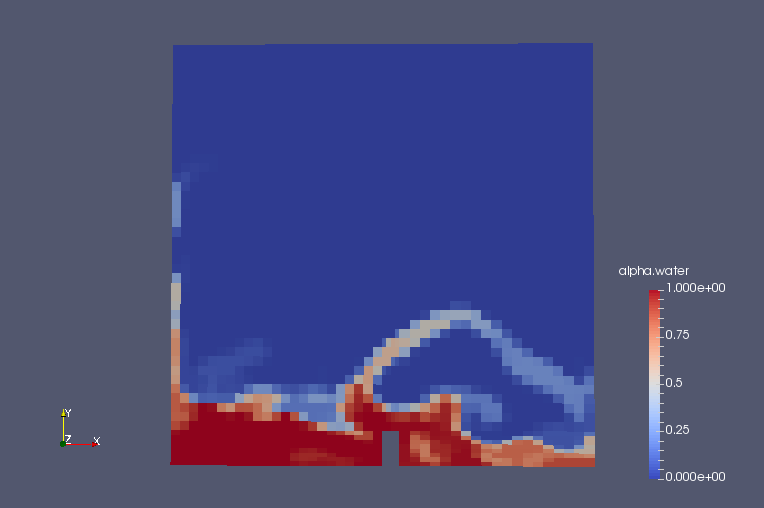﻿ Dam-break problem (Laminar) - XSim

# Dam-break problem (Laminar)

Update: July 1, 2017
OpenFOAM 4.x

## Case directory

\$FOAM_TUTORIALS/multiphase/interFoam/laminar/damBreak

## SummaryModel geometry

The dam-break problem (collapse of the water column) is calculated for 1 second. The ceiling of the analysis domain (region "atmosphere") is assumed to be open, and the left wall, right wall and floor are assumed to be under no-slip conditions. The calculation is performed as a two-dimensional problem with a single mesh in the Z direction.

The direction of gravity acceleration is defined in the file "constant/g" as follows.

```dimensions      [0 1 -2 0 0 0 0];
value           (0 -9.81 0);
```

In the file "constant/turbulenceProperties", we set that the turbulence model should not be used in the calculation by setting the following.

```simulationType  laminar;
```

The meshes are as follows, and the number of mesh is 2268.MeshesMeshes (front view)

The calculation result is as follows.

Volume ratio of water (alpha.water)Volume ratio of water at initial time (alpha.water)Volume ratio of water at final time (alpha.water)

## Commands

cp -r \$FOAM_TUTORIALS/multiphase/interFoam/laminar/damBreak damBreak
cd damBreak/damBreak

blockMesh
setFields
interFoam

paraFoam

## Calculation time

8.49 seconds *Single, Inter(R) Core(TM) i7-2600 CPU @ 3.40GHz 3.40GHz